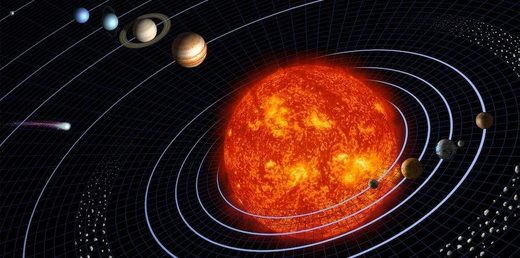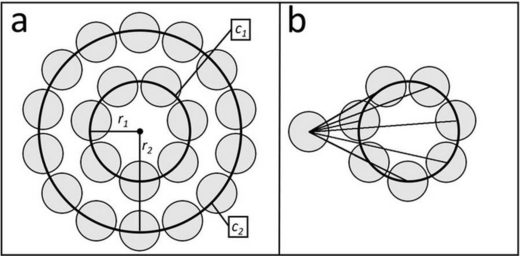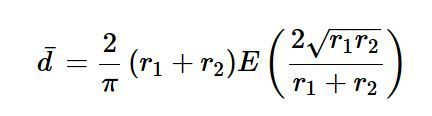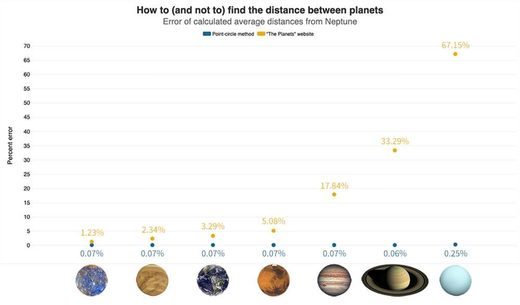An illustration of the solar system.
Calculations and simulations confirm that on average, Mercury is the nearest planet to Earth-and to every other planet in the solar system.

Quick: Which planet is closest to Earth? Ask an astronomer or a search engine, and you'll probably hear that though the situation changes frequently, Venus is the closest when averaged over time. Several educational websites, such as The Planets and Space Dictionary, publish the distance between each pair of planets, and they all show that Venus is nearest to Earth on average. They're all wrong. NASA literature even tells us Venus is "our closest planetary neighbor," which is true if we are talking about which planet has the closest approach to Earth but not if we want to know which planet is closest on average.

As it turns out, by some phenomenon of carelessness, ambiguity, or groupthink, science popularizers have disseminated information based on a flawed assumption about the average distance between planets. Using a mathematical method that we devised, we determine that when averaged over time, Earth's nearest neighbor is in fact Mercury.

That correction is relevant to more than just Earth's neighbors. The solution can be generalized to include any two bodies in roughly circular, concentric, and coplanar orbits. By using a more accurate method for estimating the average distance between two orbiting bodies, we find that this distance is proportional to the relative radius of the inner orbit. In other words, Mercury is closer to Earth, on average, than Venus is because it orbits the Sun more closely. Further, Mercury is the closest neighbor, on average, to each of the other seven planets in the solar system.

Simple but wrong

To calculate the average distance between two planets, The Planets and other websites assume the orbits are coplanar and subtract the average radius of the inner orbit, r1, from the average radius of the outer orbit, r2. The distance between Earth (1 astronomical unit from the Sun) and Venus (0.72 AU) comes out to 0.28 AU. The table at the bottom of the article shows the calculated distance between each pair of planets using that method.

Although it feels intuitive that the average distance between every point on two concentric ellipses would be the difference in their radii, in reality that difference determines only the average distance of the ellipses' closest points. Indeed, when Earth and Venus are at their closest approach, their separation is roughly 0.28 AU-no other planet gets nearer to Earth. But just as often, the two planets are at their most distant, when Venus is on the side of the Sun opposite Earth, 1.72 AU away. We can improve the flawed calculation by averaging the distances of closest and farthest approach (resulting in an average distance of 1 AU between Earth and Venus), but finding the true solution requires a bit more effort.

A better approach

To more accurately capture the average distance between planets, we devised the point-circle method. The PCM treats the orbits of two objects as circular, concentric, and coplanar. For our solar system, that's a pretty reasonable assumption: The eight planets have an average orbital inclination of 2.6° ± 2.2°, and the average eccentricity is 0.06 ± 0.06. An object in a circular orbit maintains constant velocity, which means that over a sufficiently long period, it is equally likely to be in any position in that orbit. We consider a planet's position at any given time as a uniform probabilistic distribution around a circle defined by the average orbital radius, as shown in figure 1a. The average distance between two planets can therefore be described as the average distance of every point on the circle c2, defined by r2, to every point on the circle c1, defined by r1.© Tom Stockman, et al
Figure 1. (a) The uniform probabilistic distribution of two circularly orbiting bodies, with orbital radii r1 and r2. (b) Radial symmetry is used to simplify the average distance between the distributions.
Due to rotational symmetry, the average distance from a particular point on c2 to every point on c1 is the same for any other point chosen on c2. Therefore, is also equivalent to the average distance of a single point on c2 to every point on c1, as shown in figure 1b. It can be determined by integrating the point-to-point distance around c1, as simplified in equation 1, which defines the PCM:where E(x) is an elliptic integral of the second kind. The average distances between pairs of planets determined by the PCM are included in the table. Venus is on average 1.14 AU from Earth, but Mercury is a much closer 1.04 AU.

Figure 2. A simulation of an Earth year's worth of orbits by the terrestrial planets begins to reveal that Mercury (gray in orbital animation) has the smallest average distance from Earth (blue) and is most frequently Earth's nearest neighbor. In addition, planetary geoscientist David Rothery ran a solar system simulation for the BBC radio program More or Less and came up with similar results
From the PCM, we noticed that the distance between two orbiting bodies is at a minimum when the inner orbit is at a minimum. That observation results in what we call the whirly-dirly corollary (named after an episode of the cartoon Rick and Morty): For two bodies with roughly coplanar, concentric, circular orbits, the average distance between the two bodies decreases as the radius of the inner orbit decreases. It's clear from this corollary, and from the table, that Mercury (average orbital radius of 0.39 AU), not Venus (average radius of 0.72 AU), is the closest planet to Earth on average. In fact, Mercury is even the closest planet to Neptune. (And yes, to Pluto too: Though the corollary doesn't work as well for the dwarf planet, with its orbital inclination of 17° and eccentricity of 0.25, its nearest neighbor is also Mercury.)

Simulation validation

We ran a simulation to confirm the whirly-dirly corollary, using a Python library called PyEphem to chart the positions of all eight planets in the solar system for 10 000 years. An animation illustrating the simulation is shown in figure 2. After each 24 hours of simulated time, the program records the distances between each pair of planets.

In the table we list the average measured distances over the 10 millennia and compare them with the results from the PCM and the traditional method. The simulation results differ from the flawed numbers by up to 300%, but they deviate from the PCM figures by less than 1%. Figure 3 compares the results from both methods with the simulation of the average distance between Neptune and the seven other planets.© Greg Stasiewicz and Flourish
Figure 3. The point-circle method (blue points) calculates the average distance from Neptune to the other seven planets far more accurately than the method used by websites such as The Planets (yellow). The values are compared with those obtained from the 10 000-year solar system simulation.
As best we can tell, no one has come up with a concept like PCM to compare orbits. With the right assumptions, PCM could possibly be used to get a quick estimate of the average distance between any set of orbiting bodies. Perhaps it can be useful for quickly estimating satellite communication relays, for which signal strength falls off with the square of distance. In any case, at least we know now that Venus is not our closest neighbor - and that Mercury is everybody's.
A comparison of the average distances (in astronomical units) between planets, as obtained via simulation, the point-circle method, and the common method used by various educational websites. The PCM results are far closer to those from the simulation, correctly demonstrating that Mercury is every other planet's closest neighbor.
Tom Stockman is a PhD candidate at the University of Alabama in Huntsville (UAH) and a graduate research assistant at Los Alamos National Laboratory (LANL). Gabriel Monroe is a research mechanical engineer at the US Army's Engineer Research Development Center (ERDC). Samuel Cordner is a mechanical engineer at NASA. The views expressed in this article do not necessarily represent the views of UAH, LANL, ERDC, NASA, or the US government. The authors would like to thank Michael Barton of a.i. solutions, who used FreeFlyer astrodynamics software to independently validate the simulation results; Andrew Heaton at NASA for validation of results and interesting insights; and Paul Fabel of Mississippi State University for valuable and entertaining discussion on the subject.